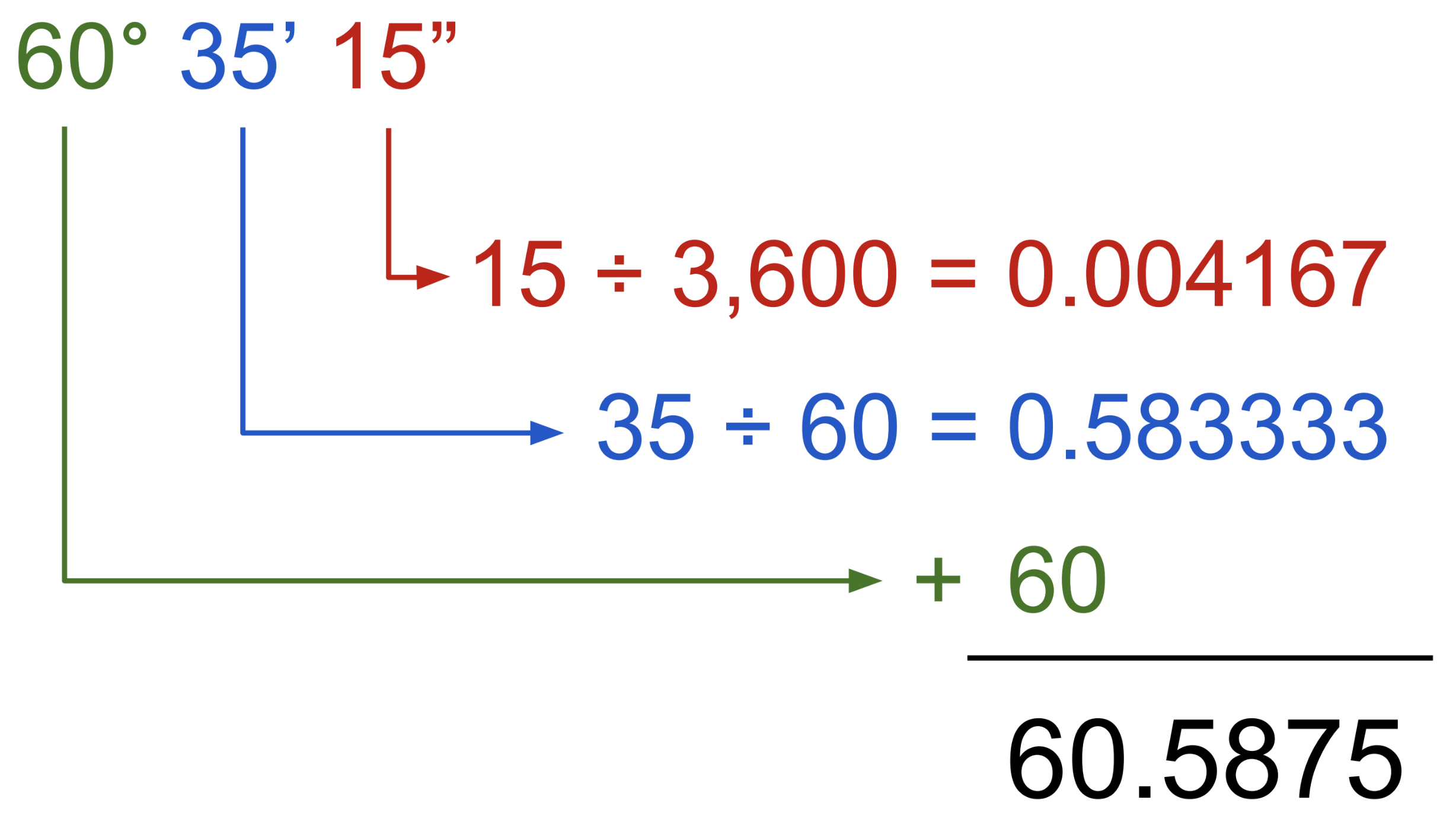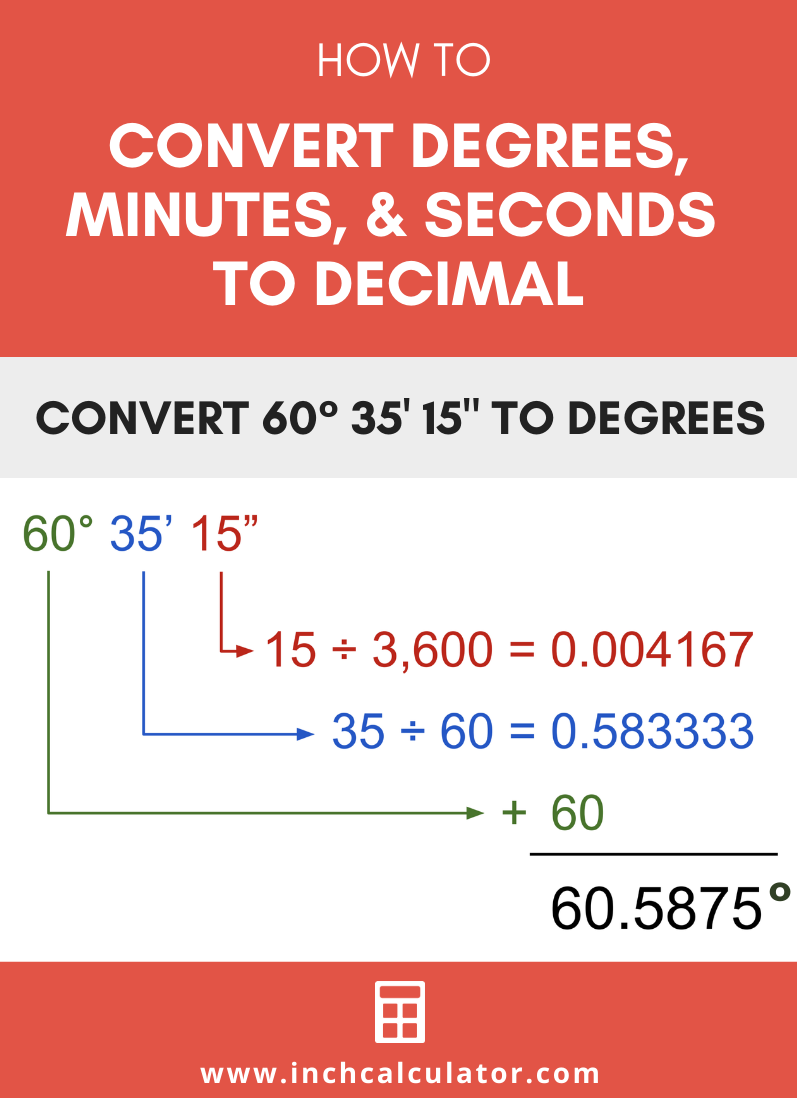# Degrees, Minutes, Seconds to Decimal Calculator

Enter degrees, minutes, and seconds to convert them to degrees in decimal form.

Angle:
°

## Result:

60.5875°
Learn how we calculated this below

## How to Convert Degrees, Minutes, Seconds (DMS) to Decimal

Degrees can be expressed with minutes and seconds or in decimal form. One degree is equal to 60 minutes or 3,600 seconds.

To convert degrees to decimal form, find the decimal value of degrees for the minutes and seconds, and then add them to the degrees.

### Step One: Convert Minutes to Decimal Degrees

Minutes are expressed using the prime symbol (′). Minutes can be converted to decimal form by dividing them by 60, since there are 60 minutes in a degree.
decimal = minutes ÷ 60

### Step Two: Convert Seconds to Decimal Degrees

Seconds are expressed using the double-prime symbol (″). Seconds can be converted to decimal form by dividing them by 3,600, since there are 3,600 seconds in a degree.
decimal = seconds ÷ 3,600

### Step Three: Add Degrees, Minutes, & Seconds

Once the minutes and seconds have been converted to degrees, the final step to convert to decimal is to add them to the whole degrees. Thus, the decimal value is equal to the whole degrees plus the converted minutes plus the converted seconds.

### Degrees, Minutes, Seconds to Decimal Formula

Summing it all up, the formula to convert DMS to decimal is:
decimal = degrees + (minutes ÷ 60) + (seconds ÷ 3,600)

For example, let’s convert 60° 35′ 15″ to decimal form.

decimal = 60 + (35′ ÷ 60) + (15″ ÷ 3,600)
decimal = 60 + 0.583333 + 0.004167
decimal = 60.5875°

Thus, 60° 35′ 15″ is equal to 60.5875°.You might also be interested in our calculator to convert decimal degrees to degrees, minutes, and seconds.